# Effect of Heat Transfer on the Growing Bubble with the Nanoparticles/Water Nanofluids in Turbulent Flow

Effect of Heat Transfer on the Growing Bubble with the Nanoparticles/Water Nanofluids in Turbulent Flow
Mathematics and Computer Science Department, Faculty of Science, Menoufia University, Shebin El-Koom 32511, Egypt

Fluid Dynamics and Seismics Lab, Moscow Institute of Physics and Technology, Dolgoprudny 141700, Moscow Region, Russia

Mechanical Engineering Department, Sreenidhi Institute of Science and Technology, Hyderabad 501301, India

Corresponding Author Email:
alibakrm@yahoo.com
Page:
95-102
|
DOI:
https://doi.org/10.18280/mmep.080112
30 November 2020
|
Accepted:
4 February 2021
|
Published:
28 February 2021
| Citation

OPEN ACCESS

Abstract:

This paper is devoted to study the effect of heat transfer on the temperature distribution in a superheated liquid during the growth of vapour bubbles immersed in different types of nanoparticles/water nanofluids between two-phase turbulent flow. The mathematical model is formulated and solved analytically depending on Scriven's theory and using the modification of the method of the similarity parameters between two finite boundaries. The characteristics of vapour bubble growth and temperature distribution are obtained by using the thermo-physical properties of nanoparticles nanofluids. The results indicate that the nanoparticle volume concentration reduces the bubble growth process under the effect of heat transfer. The better agreements are achieved, for bubble dynamics in turbulent nanofluid using the appropriate numerical and theoretical data for the values of concentration rate of nanoparticles χ=0,0.2,0.4. The temperature distribution surrounding the regime of bubble growth in pure water is more intensive than in other cases of Al2O3/H2O, Fe3O4/H2O and CuO/H2O nanofluids in turbulent flow. A Comparison of the current solution with previous works is carried out and discussed.

Keywords:

temperature distribution, bubble growth, nanoparticles/water nanofluids, turbulent flow, mathematical modeling

1. Introduction

The field of nanofluids has novel properties of the fluid that make intensively useful in the field of heat transfer and its application including refrigerators, fuel cells, heat exchanger in the systems and conventional colloids [1-6]. It is appeared in the properties of thermal conductivity and convective heat/mass transfer. Measurably, the nanofluids have happened when the scale of measurement is smaller than 100nm functionalized nanoparticles, it is considered that a new class of material. It is known that the efficiency of heat transfer enhancement depends on the number of particles distributed, the type of the substance, the shape of particles, and so on. Bubble dynamics and nanofluids are planned to be used in aircraft, engines, micro-reactors [5, 6]. Prior to the introduction of nanofluids, it was expected that heat transfer could be enhanced by dispersing micron-sized particles. But the fluid of micron-sized particles caused problems due to sedimentation and clogging.

A lot of research has undergone for the last years in the field of boiling systems of nanofluids. However, for most potential applications of nanofluids the situation of interest is flow boiling including bubble dynamics parameters, such as bubble departure diameter, frequency and void fraction during nanofluid flow boiling, that may provide valuable insight in the mechanisms by which the nanoparticles affect the heat transfer .

Recently, it has become increasingly necessary to study various methods for increasing heat transfer especially in the field of bubble dynamics and their applications like peristaltic motion  and nuclear power system , resulting from the transfer of nanoparticles in common liquids called nanofluids. Measurements of nanoparticles are used in nanofluids range from 1:100 nm. These nanoparticles are metal particles such as Cu, Ag or other metal oxides. Typical fluids used in heat transfer usually have a low coefficient of heat transfer, but due to their high coefficient of conductivity, the use of nanoparticles can increase the coefficient of thermal conductivity of mixing fluids. The use of nanofluids is also a suitable approach to improve heat transfer [6, 8-10]. The distribution of temperature around the growth of a vapour bubble is an important process in nanoparticles/water (for example, Al2O3/H2O, Fe3O4/H2O and CuO//H2O) nanofluids between a two-phase turbulent flow. In nanoparticles nanofluid, the growth process is influenced by heat transfer and pressure change between the laminar or turbulent flow of two phases. The problem of heat transfer in a growing bubble with initial conditions in the laminar flow is introduced and discussed in Ref. .

The bubble dynamics in nanofluids are studied experimentally and introduced by some efforts of researchers [5, 12-15]. Park et al.  attempted to formulate the problem of bubble dynamics in nanofluids and find experimentally the behaviours of boiling bubbles. Moreover single bubble is studied in Al2O3/H2, Fe3O4/H2O and CuO//H2O nanofluids considering the impact of heating surface. In another side, some works (as in Refs. [16, 17] showed the model of the bubble dynamics in viscoelastic fluids that took Oldroyd constitutive equation on works [16, 17], which allows for strain relaxation of the fluid and is more suitable to greater deformations. The evaluation of radius under influence of shear stress is theoretically studied in ref. [18, 19]. Recently, Abu-Nab et al.  investigated the vapour bubble dynamic in power-law Al2O3/H2 nanofluid, affecting by variable surface tension depending on the method of Plesset and Zwick  and Mohammadein et al. . Furthermore, the effects of surface tension behavior with the initial bubble radius, time, and initial rate of bubble radius are investigated within Al2O3/H2 nanofluid. It is found that the growth of the vapour bubble radius is affected by the concentration rate of nanoparticles and other parameters. However, the influence of heat transfer on growth process of bubbles in nanoparticles/water nanofluids between two-phase turbulent flow has not been studied analytically before. Consequently, we will take that into account.

The main aim of the current work is to study the effect of heat transfer on a growing bubble in Al2O3/H2, Fe3O4/H2O and CuO//H2O nanofluids between two-phase turbulent flow and determine the bubble radius under the influence of thermo-physical parameters of superheating liquid, nanoparticles volume fraction and density in nanoparticles/water nanofluids. Besides, we illustrate the temperature distribution in the regime of bubble growth in turbulent flow, during nanoparticles/water nanofluids.

The introduction presented in section one. The theoretical study, mathematical model and solution of the model are introduced in section two and three. Results and discussions, and conclusion are addressed in section four and five respectively.

2. Theoretical Model and Mathematical Formulation

As is known, a single vapor bubble grows between two boundaries of a finite radius $\mathrm{R}_{0}$ and $\mathrm{R}_{\mathrm{m}}$ inside a superheated incompressible liquid (as shown in Figure 1) with different patterns of nanoparticles/water nanofluids like Al2O3/H2, Fe3O4/H2O and CuO//H2O. The single bubble has a spherical geometry. The growth is influenced by the pressure difference between the internal pressure of the bubble $(\mathrm{P}(\mathrm{v}),(\mathrm{R}(\mathrm{t}), \mathrm{t})$ and heat transfer in different types of nanoparticles nanofluids between two-phase turbulent flow. Steam and superheated liquid are considered incompressible flows. The pressure inside the bubble is uniform and also the distribution of vapor density inside the bubble is uniform, with the exception of a thin boundary layer at the bubble wall. The thermo-physical properties of particles nanofluids are considered and presented into account in the presented model.

We suppose that the bubble velocity in a laminar flow is greater than the fluctuation velocity for turbulent flow. The equation between them, had been assumed in ref.  as

$\dot{\mathrm{R}}_{\mathrm{Lam}}=\mathrm{n} \dot{\mathrm{R}}_{\mathrm{Tur}}$        (1)

here, n is the arbitrary constant; $\dot{\mathrm{R}}_{\text {Lam }}, \dot{\mathrm{R}}_{\mathrm{Tur}}$ are bubble velocity in laminar and turbulent flow respectively. In the case of study bubble dynamics in turbulent flow, the condition constraint, n≥1 is satisfied.

The primary mathematical model describing the present problem of effect of heat transfer on bubble dynamics with different types of nanofluids between a two-phase turbulent flow, consists of mass, energy equations and the relation of the thermo-physical properties of particles nanofluids.

## 1.pngFigure 1. Schematic of a growing vapour bubble immersed in some different types of nanoparticles/water nanofluids between two-phase temperatures

2.1 Mass equation

The continuity equation in an incompressible liquid  can be expressed as:

$\operatorname{div} \mathbf{V}=0$           (2)

where, $\mathbf{V}=\mathbf{V}_{\text {Lam }}+\mathbf{V}_{\text {Tur }} ; \quad \mathbf{V}_{\text {Lam }}, \mathbf{V}_{\text {Tur }}$ are the velocity of mixture in laminar flow and turbulent flow respectively.

Assuming the initial and boundary conditions: $(\mathrm{t}=0, \mathrm{r}=$ $\left.\mathrm{R},\left(\mathrm{V}_{\mathrm{Lam}}\right)_{\mathrm{r}=\mathrm{R}}=\varepsilon_{\mathrm{nf}} \mathrm{a} \dot{\mathrm{R}}_{\mathrm{Lam}},\left(\mathrm{V}_{\mathrm{Tur}}\right)_{\mathrm{r}=\mathrm{R}}=\varepsilon_{\mathrm{nf}} \mathrm{b} \dot{\mathrm{R}}_{\mathrm{Tur}}\right) ;$ where $\varepsilon_{\mathrm{nf}}=1-\frac{\rho_{\mathrm{v}}}{\left(\rho_{1}\right) \mathrm{nf}}$ is the density ratio in nanofluids; $\rho_{\mathrm{v}}$ and $\left(\rho_{\mathrm{l}}\right)_{\mathrm{nf}}$ are the density of vapour and nanofluid respectively. a,b are arbitrary constants. Using the above assumptions and Eq. (1) to solve the continuity Eq. (2), then the mixture velocity can be solved as:

$V=\frac{\varepsilon_{\mathrm{nf}} C_{\mathrm{k}} \mathrm{R}^{2}}{\mathrm{r}^{2}} \dot{\mathrm{R}}$       (3)

Here Ck=a+bn. The arbitrary parameter $\mathrm{C}_{\mathrm{k}}$ plays a dominant parameter to determine the kind of the flow on bubble dynamic process. That means, if Ck<1, the flow is in turbulent flow, and if Ck≥1, the flow is in laminar flow.

2.2 Energy equation

The energy equation is extended for spherical symmetry to establish the effect of radial conduction resulting from unequal phase temperature. The energy equation in turbulent flow (see for example ) can be rewritten in the form

$\frac{\partial \mathrm{T}}{\partial \mathrm{t}}+\mathrm{V} \frac{\partial \mathrm{T}}{\partial \mathrm{t}}=\mathrm{c}_{0}^{2} \mathrm{a}_{\mathrm{Tur}} \mathrm{r}^{2} \frac{\partial}{\partial \mathrm{r}}\left(\mathrm{r}^{2} \frac{\partial \mathrm{T}}{\partial \mathrm{r}}\right)$          (4)

Here $\mathrm{a}_{\mathrm{Tur}}$ is the thermal diffusivity in turbulent flow and c0 can be defined in ref.  as $c_{0}=\left(2.2 \times 10^{-3} \mathrm{P}_{\mathrm{e}}\right)^{-1 / 2}$; Pe is the relation among the thermal energy related to the fluid and the thermal energy conducted within the fluid, is called the Péclet number,

The Initial conditions and boundary conditions can be expressed as

$\mathrm{T}(\mathrm{R}, 0)=\mathrm{T}_{\mathrm{s}}, \quad \mathrm{T}\left(\mathrm{R}_{\mathrm{m}}, \mathrm{t}\right)=\mathrm{T}_{0},\left.\frac{\partial \mathrm{T}}{\partial \mathrm{r}}\right|_{\mathrm{r}=\mathrm{R}}=\frac{\varepsilon_{\mathrm{nf}} \mathrm{C}_{\mathrm{k}} \rho_{\mathrm{v}}\left(\mathrm{L}+\left(\left(\mathrm{C}_{\mathrm{P}}\right)_{\mathrm{nf}}-\mathrm{C}_{\mathrm{Pv}}\right) \Delta \Omega\right) \dot{\mathrm{R}}}{\mathrm{k}_{\mathrm{nf}}}$        (5)

Here, Ts is saturation temperature of bubble, T0 is initial temperature of liquid. (Cp)nf and CPv are specific heat of nanofluids and vapour respectively, L is a latent heat vaporization, Knf is liquid thermal conduction in nanofluid and ΔΩ is superheating temperature of bulk liquid.

2.3 Thermo-physical properties of nanoparticles/water nanofluids

In the study of bubble dynamics in nanofluids, the main key thermo-physical properties of nanofluids are to investigate the properties of density, specific heat capacity, thermal conductivity and surface tension during the bubble dynamic process. The thermo-physical relations (see for examples, refs. [5, 9, 26, 27]) describe the problem of heat transfer on a growing vapor bubble between a two-phase turbulent flow has the following form as

$\rho_{\mathrm{nf}}=(1-\chi) \rho_{\mathrm{f}}+\chi \rho_{\mathrm{p}}$         (6a)

$\left(c_{p}\right)_{n f}=(1-\chi)\left(c_{p}\right)_{f}+\chi\left(c_{p}\right)_{p}$        (6b)

$k_{n f}=\frac{\left(k_{p}+2 k_{f}\right)-2 x\left(k_{p}-k_{f}\right)}{\left(k_{p}+2 k_{f}\right)+\chi\left(k_{p}-k_{f}\right)} k_{f}$        (6c)

where, $\rho_{\mathrm{f}}, \rho_{\mathrm{p}}$ are the density of fluid and particle respectively. $\chi$ is the concentration rate of nanoparticle in nanofluids. The index nf indicates to nanofluid. (Cp)f, (Cp)P are the specific heat of fluid and particle respectively. kf, kp are thermal conductivity of fluid and particle respectively.

Eq. (6a) represents the equation of density in nanofluid. Equation (6b) represents the equation of specific heat in nanofluid. Eq. (6c) is the relation of thermal conductivity in nanofluids, is used to in ref. , in our model, we consider that the effects of the Brownian motion are negligible due to the randomly large particles at a low concentration of nanoparticles. The thermo-physical properties of pure water and some nanoparticles are illustrated in Table 1. These thermo-physical properties are used into presented account.

Table 1. Thermo-physical properties of water based copper oxide, iron oxide and alumina nanofluids [26, 28, 29]

 Properties Base Fluid CuO $\mathbf{A l}_{2} q\mathbf{O}_{3}$ $\mathbf{F e}_{3} \mathbf{O}_{4}$ $\mathrm{C}_{\mathrm{p}}(\mathrm{J} / \mathrm{kg} \mathrm{K})$ 4179 531.8 765 670 $\rho\left(\mathrm{kg} / \mathrm{m}^{3}\right)$ 997.1 6320 3970 5180 $\mathrm{k}_{\mathrm{l}}(\mathrm{W} / \mathrm{m} \mathrm{K})$ 0.613 76.5 40 9.7
3. Solution of the Model

This section is to present the solution of theoretical model of the temperature distribution surrounding the growing bubbles in some different types of nanoparticles/water nanofluids, based on the reduced system of Eqns. (1-6) and the method of the similarity parameter between two finite boundaries, we will use the following as

$\mathrm{T}(\mathrm{r}, \mathrm{t})=\mathrm{T}(\mathrm{s}), \mathrm{r}=\frac{1}{\beta} \mathrm{s} \mathrm{f}(\mathrm{t})$ and $\frac{\partial \mathrm{s}}{\partial \mathrm{t}}=0$          (7)

At the boundary of bubble, we suppose r=R then

s=β             (8)

And r=f(t).

The temperature gradient in the mixture of nanofluids at boundary of bubble takes

$4 \pi \mathrm{R}^{2} \mathrm{k}_{\mathrm{nf}}\left(\frac{\partial \mathrm{T}}{\partial \mathrm{r}}\right)_{\mathrm{r}=\mathrm{R}}=\frac{\mathrm{d}}{\mathrm{dt}}\left[\frac{4 \pi \mathrm{R}^{3}}{3}\left(\mathrm{~L}+\left(\left(\mathrm{C}_{\mathrm{P}}\right)_{\mathrm{nf}}-\mathrm{C}_{\mathrm{Pv}}\right) \Delta \Omega\right) \rho_{\mathrm{v}}\right]$          (9)

Combining (3) and (9), the temperature gradient $\left(\frac{\partial \mathrm{T}}{\partial \mathrm{r}}\right)_{\mathrm{r}=\mathrm{R}}$ becomes

$\left(\frac{\partial \mathrm{T}}{\partial \mathrm{r}}\right)_{\mathrm{r}=\mathrm{R}}=\frac{\varepsilon_{\mathrm{nf}} \mathrm{C}_{\mathrm{k}} \rho_{\mathrm{v}}}{\mathrm{k}_{\mathrm{nf}}} \dot{\mathrm{R}}\left(\mathrm{L}+\left(\left(\mathrm{C}_{\mathrm{P}}\right)_{\mathrm{nf}}-\mathrm{C}_{\mathrm{Pv}}\right) \Delta \Omega\right)$        (10)

On the basis of the above assumptions and applying the above assumptions and separating of variables methods on

Eq. (4), then the energy Eq. (4) converts to two equations, the obtained equation can be divided to two differential equations as

$\dot{\mathrm{f}}(\mathrm{t}) \mathrm{f}(\mathrm{t})=\mathcal{A}$            (11)

And

$\frac{\mathrm{d}}{\mathrm{ds}} \ln \left(\frac{\mathrm{dT}}{\mathrm{ds}}\right)=\frac{\mathcal{A}}{\mathrm{a}_{\mathrm{Tur}} \mathrm{c}_{0}^{2}}\left[-\frac{\mathrm{s}}{\beta^{2}}+\frac{\varepsilon_{\mathrm{nf}} \mathrm{C}_{\mathrm{k}} \beta}{\mathrm{s}^{2}}\right]-\frac{2}{\mathrm{~s}}$          (12)

We integrate Eq. (12), we get

$\frac{\mathrm{dT}}{\mathrm{ds}}=\frac{\mathcal{A}}{\mathrm{s}^{2}} \mathrm{e}^{\left[\frac{-\mathcal{A}}{\mathrm{a}_{\mathrm{Tur}} \mathrm{c}_{0}^{2}}\left(\frac{\mathrm{s}^{2}}{2 \beta^{2}}+\frac{\varepsilon_{\mathrm{nf}} \mathrm{C}_{\mathrm{k}} \beta}{\mathrm{s}}\right)\right]}$        (13)

The boundary condition (5) can be converted to

$\left(\frac{\mathrm{dT}}{\mathrm{dr}}\right)_{r=\mathrm{R}}=\frac{\mathcal{A} \varepsilon_{\mathrm{nf}} \mathrm{C}_{\mathrm{k}} \rho_{\mathrm{v}}\left(\mathrm{L}+\left(\left(\mathrm{C}_{\mathrm{P}}\right)_{\mathrm{nf}}-\mathrm{C}_{\mathrm{Pv}}\right) \Delta \Omega\right)}{\mathrm{k}_{\mathrm{n} \mathrm{R}} \mathrm{R}}$      (14)

We obtain $\frac{\mathrm{dT}}{\mathrm{dr}}=\frac{\mathrm{s}}{\mathrm{r}} \frac{\partial \mathrm{T}}{\partial \mathrm{s}}$, thus

$\left(\frac{\mathrm{dT}}{\mathrm{ds}}\right)_{\mathrm{r}=\beta}=\left(\frac{\mathrm{r}}{\mathrm{s}} \frac{\mathrm{dT}}{\mathrm{dr}}\right)_{\mathrm{r}=\mathrm{R}, \mathrm{s}=\beta}=\frac{\mathcal{A} \varepsilon_{\mathrm{nf}} \mathrm{C}_{\mathrm{k}} \rho_{\mathrm{v}}\left(\mathrm{L}+\left(\left(\mathrm{C}_{\mathrm{P}}\right)_{\mathrm{nf}}-\mathrm{C}_{\mathrm{Pv}}\right) \Delta \Omega\right)}{\mathrm{k}_{\mathrm{nf}} \beta}$           (15)

To find the constant $\mathcal{A}_{1}$, using (15) into (13), then

\begin{aligned} \mathcal{A}_{1}=\frac{1}{\mathrm{k}_{\mathrm{nf}}} \mathcal{A} \varepsilon_{\mathrm{nf}} \mathrm{C}_{\mathrm{k}} \rho_{\mathrm{v}}(\mathrm{L}+\left(\left(\mathrm{C}_{\mathrm{P}}\right)_{\mathrm{nf}}\right.\left.\left.-\mathrm{C}_{\mathrm{Pv}}\right) \Delta \Omega\right) \beta \mathrm{e}\left[\frac{\mathcal{A}}{\mathrm{a}_{\mathrm{Tur}} c_{0}^{2}}\left(\frac{1}{2}+\varepsilon_{\mathrm{nf}} \mathrm{C}_{\mathrm{k}}\right)\right] \end{aligned}

Using the value of constant $\mathcal{A}_{1}$ into Eq. (13), we get

$\frac{\mathrm{dT}}{\mathrm{ds}}=\frac{\mathcal{A}_{2} \mathcal{A} \beta}{\mathrm{s}^{2}} \mathrm{e}^{\left[\frac{-\mathcal{A}}{\mathrm{a}_{\mathrm{Tur}} \mathrm{c}_{0}^{2}}\left(\frac{\mathrm{s}^{2}}{2 \beta^{2}}+\frac{\varepsilon_{\mathrm{nf}} \mathrm{C}_{\mathrm{k}} \beta}{\mathrm{s}}\right)+\frac{\mathcal{A}}{\mathrm{a}_{\mathrm{Tur}} \mathrm{c}_{0}^{2}}\left(\frac{1}{2}+\varepsilon_{\mathrm{nf}} \mathrm{C}_{\mathrm{k}}\right)\right]}$          (16)

where, $\mathcal{A}_{2}=\frac{1}{\mathrm{k}_{\mathrm{nf}}} \varepsilon_{\mathrm{nf}} \mathrm{C}_{\mathrm{k}} \rho_{\mathrm{v}}\left(\mathrm{L}+\left(\left(\mathrm{C}_{\mathrm{P}}\right)_{\mathrm{nf}}-\mathrm{C}_{\mathrm{Pv}}\right) \Delta \Omega\right)$.

From Eq. (15), Eq. (16) leads to

$\frac{\partial \mathrm{T}}{\partial \mathrm{r}}=\frac{\mathrm{s}}{\mathrm{r}} \frac{\mathrm{dT}}{\mathrm{ds}}$

$=\frac{\mathcal{A}_{2} \mathcal{A} \beta}{\mathrm{r} \mathrm{s}} \mathrm{e}^{\left[\frac{-\mathcal{A}}{\mathrm{a}_{\mathrm{Tur}} \mathrm{c}_{0}^{2}}\left(\frac{\mathrm{s}^{2}}{2 \beta^{2}}+\frac{\varepsilon_{\mathrm{nf}} \mathrm{C}_{\mathrm{k}} \beta}{\mathrm{s}}\right)+\frac{\mathcal{A}}{\mathrm{a}_{\mathrm{Tur}} \mathrm{c}_{0}^{2}}\left(\frac{1}{2}+\varepsilon_{\mathrm{nf}} \mathrm{C}_{\mathrm{k}}\right)\right]}$          (17)

Using the assumptions in (7,8), Eq. (17) becomes

$\frac{\partial \mathrm{T}}{\partial \mathrm{r}}=\frac{\mathcal{f}_{2} \mathcal{A} \mathrm{R}}{\mathrm{r}^{2}} \mathrm{e}^{\left[\frac{-\mathcal{A}}{\mathrm{a}_{\mathrm{Tur}} \mathrm{c}_{0}^{2}}\left(\frac{\mathrm{r}^{2}}{2 \mathrm{R}^{2}}+\frac{\varepsilon_{\mathrm{nf}} \mathrm{C}_{\mathrm{k}} \mathrm{R}}{\mathrm{r}}\right)+\frac{\mathcal{A}}{\mathrm{a}_{\mathrm{Tur}} \mathrm{c}_{0}^{2}}\left(\frac{1}{2}+\varepsilon_{\mathrm{nf}} \mathrm{C}_{\mathrm{k}}\right)\right]}$             (18)

Eq. (18) can be integrated from any instant $\mathrm{t}$ to $\mathrm{t}_{\mathrm{m}}$ at $\mathrm{r}$ reaches to maximum radius Rm also T(r, t)=T0, that means

$\mathrm{T}(\mathrm{r}, \mathrm{t})=\mathrm{T}_{0}-\mathcal{A}_{2} \mathcal{A}$

$\mathrm{R} \int_{\mathrm{r}}^{\mathrm{R}_{\mathrm{m}}} \frac{1}{\mathrm{z}^{2}} \mathrm{e}^{\left[\frac{-\mathcal{A}}{\mathrm{a}_{\mathrm{Tur}} \mathrm{c}_{0}^{2}} \cdot\left(\frac{\mathrm{z}^{2}}{2 \mathrm{R}^{2}}+\frac{\varepsilon_{\mathrm{nf}} \mathrm{C}_{\mathrm{k}} \mathrm{R}}{\mathrm{z}}\right)+\frac{\mathcal{A}}{\mathrm{a}_{\mathrm{Tur}} \mathrm{c}_{0}^{2}} \cdot\left(\frac{1}{2}+\varepsilon_{\mathrm{nf}} \mathrm{C}_{\mathrm{k}}\right)\right]} \mathrm{dz}$           (19)

In the wall of bubble, we suppose r=R(ti), Eq. (19) becomes

$\mathrm{T}\left(\mathrm{R}\left(\mathrm{t}_{\mathrm{i}}\right), \mathrm{t}_{\mathrm{i}}\right)=\mathrm{T}_{0}-\mathcal{A}_{2} \mathcal{A} \cdot \mathrm{R}\left(\mathrm{t}_{\mathrm{i}}\right)$

$\int_{\mathrm{R}\left(\mathrm{t}_{\mathrm{i}}\right)}^{\mathrm{R}_{\mathrm{m}}} \frac{1}{\mathrm{z}^{2}} \mathrm{e}^{\left[\frac{-\mathcal{A}}{\mathrm{a}_{\mathrm{Tur}} c_{0}^{2}} \cdot\left(\frac{\mathrm{z}^{2}}{2 \mathrm{R}^{2}\left(\mathrm{t}_{\mathrm{i}}\right)}+\frac{\varepsilon_{\mathrm{nf}} \mathrm{C}_{\mathrm{k}} \mathrm{R}\left(\mathrm{t}_{\mathrm{i}}\right)}{\mathrm{z}}\right)+\frac{\mathcal{A}}{\mathrm{a}_{\mathrm{Tur}} \mathrm{c}_{0}^{2}} \cdot\left(\frac{1}{2}+\varepsilon_{\mathrm{nf}} \mathrm{C}_{\mathrm{k}}\right)\right]} \mathrm{dz}$         (20)

Supposing that y=z/R(ti) into Eq. (20), we get the temperature distribution of a growing bubble in nanoparticles nanofluids between two-phase flow in the form

$\mathrm{T}\left(\mathrm{R}\left(\mathrm{t}_{\mathrm{i}}\right), \mathrm{t}_{\mathrm{i}}\right)=\mathrm{T}_{0}-\mathcal{A}_{2} \mathcal{A} \int_{1}^{\frac{\mathrm{R}_{\mathrm{m}}}{\mathrm{R}\left(\mathrm{t}_{\mathrm{i}}\right)}} \frac{1}{\mathrm{y}^{2}} \mathrm{e}^{\left[\frac{-\mathcal{A}}{\mathrm{a}_{\mathrm{Tur}} c_{0}^{2}}\left(\frac{\mathrm{y}^{2}}{2}+\frac{\varepsilon_{\mathrm{nf}} \mathrm{C}_{\mathrm{k}}}{\mathrm{y}}\right)+\frac{\mathcal{A}}{\mathrm{a}_{\mathrm{Tur}} \mathrm{c}_{0}^{2}}\left(\frac{1}{2}+\varepsilon_{\mathrm{nf}} \mathrm{C}_{\mathrm{k}}\right)\right]} \mathrm{dy}$       (21)

By integrating the R. H. S of above equation, Eq. (21) becomes

$\mathrm{T}\left(\mathrm{R}\left(\mathrm{t}_{\mathrm{i}}\right), \mathrm{t}_{\mathrm{i}}\right)=\mathrm{T}_{0}-\mathcal{A}_{2}\left(\frac{\pi}{6} \mathrm{a}_{\mathrm{Tur}} \mathrm{c}_{0}^{2} \mathcal{A}\right)^{\frac{1}{2}} \mathrm{e}^{\frac{1}{6}\frac{A\left(1-\varepsilon_{n f} C_{k}\right)^{2}}{a_{\operatorname{Tur}} c_{0}^{2}}}$

$\left(\operatorname{erf}\left[\left(\frac{3}{2} \frac{\mathcal{A}}{\mathrm{a}_{\mathrm{Tur}} \mathrm{c}_{0}^{2}}\right)^{\frac{1}{2}}\left(1-\frac{\mathrm{R}\left(\mathrm{t}_{\mathrm{i}}\right)}{\mathrm{R}_{\mathrm{m}}}\right)\right.\right. \left.+\left(1-\varepsilon_{\mathrm{nf}} C_{\mathrm{k}}\right)\left(\frac{1}{6} \frac{\mathrm{A}}{\mathrm{a}_{\mathrm{Tur}} \mathrm{c}_{0}^{2}}\right)^{\frac{1}{2}}\right] \left.-\operatorname{erf}\left[\left(1-\varepsilon_{\mathrm{nf}} \mathrm{C}_{\mathrm{k}}\right)\left(\frac{1}{6} \frac{\mathrm{c}}{\mathrm{a}_{\mathrm{Tur}} \mathrm{c}_{0}^{2}}\right)^{\frac{1}{2}}\right]\right)$        (22)

At $\mathrm{t}=\mathrm{t}_{0}, \mathrm{R}\left(\mathrm{t}_{0}\right)=\mathrm{R}_{0} \Rightarrow \operatorname{erf}(0)=0$ and $\mathrm{T}\left(\mathrm{R}\left(\mathrm{t}_{0}\right), \mathrm{t}_{0}\right)=\mathrm{T}_{\mathrm{s}},$ Eq. (22) converts to

$\Delta \Omega=\mathrm{T}_{0}-\mathrm{T}_{\mathrm{s}}=\mathcal{A}_{2}\left(\frac{\pi}{6} \mathrm{a}_{\mathrm{Tur}} \mathrm{c}_{0}^{2} \mathcal{A}\right)^{\frac{1}{2}} \operatorname{erf}\left[\left(\frac{3}{2} \frac{\mathcal{A}}{\mathrm{a}_{\mathrm{Tur}} \mathrm{c}_{0}^{2}}\right)^{\frac{1}{2}}\left(1-\phi_{0}^{1 / 3}\right)\right]$          (23)

Here $\phi_{0}=\left(R_{0} / R_{m}\right)^{3}$, is a void fraction. Ts is the saturation temperature.

Using the value of $\mathcal{A}_{2}$ into Eq. (23), we get

$\Delta f=\frac{1}{\mathrm{k}_{\mathrm{nf}}} \varepsilon_{\mathrm{nf}} \mathrm{C}_{\mathrm{k}} \rho_{\mathrm{V}}(\mathrm{L}\left.+\left(\left(\mathrm{C}_{\mathrm{P}}\right)_{\mathrm{nf}}-\mathrm{C}_{\mathrm{Pv}}\right) \Delta \Omega\right)\left(\frac{\pi}{6} \mathrm{a}_{\mathrm{Tur}} \mathrm{c}_{0}^{2} \mathcal{A}\right)^{\frac{1}{2}} \operatorname{erf}\left[\begin{array}{l}\left(\frac{3}{2} \frac{\mathrm{A}}{\mathrm{a}_{\mathrm{Tur}} \mathrm{c}_{0}^{2}}\right)^{\frac{1}{2}} \\ \left(1-\phi_{0}^{1 / 3}\right)\end{array}\right]$          (24)

We note that, $\operatorname{erf}(\mathrm{x})=1-\frac{1}{\sqrt{\pi} \mathrm{x}} \mathrm{e}^{-\mathrm{x}^{2}}$ at any $\mathrm{x} \gg 0,$ also, for

any $\mathrm{a}_{\mathrm{Tur}} \ll 1,$ and for any times, we obtain $0<\phi_{0}^{1 / 3}<1$. Then Eq. (24) reduces

$\frac{\left(J_{\mathrm{a}}\right)_{\mathrm{nf}}\left(\mathrm{a}_{\mathrm{Tur}}\right)_{\mathrm{nf}}}{\varepsilon_{\mathrm{nf}} \mathrm{C}_{\mathrm{k}}\left(1+\frac{\left(\left(\mathrm{C}_{\mathrm{P}}\right)_{\mathrm{nf}}-\mathrm{C}_{\mathrm{Pv}}\right)}{\mathrm{L}} \Delta \Omega\right.} =\left(\frac{\pi}{6} \mathrm{a}_{\mathrm{Tur}} \mathrm{c}_{0}^{2} \mathcal{A}\right)^{\frac{1}{2}}-\frac{1}{3\left(1-\phi_{0}^{\frac{1}{3}}\right)} \mathrm{e}^{\left[\left(\frac{-3}{2 \mathrm{a}_{\mathrm{Tur}} c_{0}^{2}}\right)^{\frac{1}{2}}\left(1-\phi_{0}^{1 / 3}\right)\right]}$            (25)

Here $\left(J_{a}\right)_{n f}=\frac{\rho_{n f}\left(a_{\text {Tur }}\right)_{\text {nf }}}{\rho_{\mathrm{v}} \mathrm{L}} \Delta \Omega$, is the Jacob number in nanofluids.

For aTur, we get

$\frac{\Delta \Omega \mathrm{k}_{\mathrm{nf}}}{\varepsilon_{\mathrm{nf}} \mathrm{C}_{\mathrm{k}} \rho_{\mathrm{v}}\left(1+\frac{\left(\left(\mathrm{C}_{\mathrm{P}}\right)_{\mathrm{nf}}-\mathrm{C}_{\mathrm{Pv}}\right)}{\mathrm{L}} \Delta \Omega\right)}=\left(\frac{\pi}{6} \mathrm{a}_{\mathrm{Tur}} \mathrm{c}_{0}^{2} \mathcal{A}\right)^{\frac{1}{2}}$

then $\mathcal{A}$ can be written as

$\mathcal{A}=\frac{6 \mathrm{a}_{\mathrm{Tur}}}{\pi}\left(\frac{\left(\mathrm{J}_{\mathrm{a}}\right)_{\mathrm{nf}}}{c_{0} \varepsilon_{\mathrm{nf}} \mathrm{C}_{\mathrm{k}}\left(1+\frac{\left(\left(\mathrm{C}_{\mathrm{P}}\right)_{\mathrm{nf}}-\mathrm{C}_{\mathrm{Pv}}\right)}{\mathrm{L}} \Delta \Omega\right)}\right)^{2}$             (26)

Apply the boundary conditions at t=t0, R=R0 in solving Eq. (12a) to get the bubble radius in nanofluids, then we get

$\mathrm{R}=\left(2 \mathcal{A}\left(\mathrm{t}-\mathrm{t}_{0}\right)+\mathrm{R}_{0}^{2}\right)^{\frac{1}{2}}$           (27)

Combining (26, 27), we get the bubble radius in nanofluids between two turbulent flow in a final form

$\mathrm{R}=2\left(\frac{3 \mathrm{a}_{\mathrm{Tur}}}{\pi}\left(\frac{\left(\mathrm{J}_{\mathrm{a}}\right)_{\mathrm{nf}}}{\mathrm{c}_{0} \varepsilon_{\mathrm{nf}} \mathrm{C}_{\mathrm{k}}\left(1+\frac{\left(\left(\mathrm{C}_{\mathrm{P}}\right)_{\mathrm{nf}}-\mathrm{C}_{\mathrm{Pv}}\right)}{\mathrm{L}} \Delta \Omega\right)}\right)^{2}\left(\mathrm{t}-\mathrm{t}_{0}\right)\right. \left.+\frac{1}{4} R_{0}^{2}\right)^{\frac{1}{2}}$               (28)

The formula (28) denotes the behaviour of vapour bubble growth between two-phase turbulent flow under conditions in (7), that affected by nanoparticles nanofluids.

4. Results and Discussions

The results of the present model and its solution are described in Figures 2-8 depending on the physical parameters in Table 1 and using the data of parameters that can be expressed as following as: $\rho_{\mathrm{v}}=0.579 \frac{\mathrm{kg}}{\mathrm{m}^{3}}, \mathrm{C}_{\mathrm{pv}}=$$2160 \frac{\mathrm{J}}{\mathrm{kg} \mathrm{K}}, \Delta \Omega=2.5 \mathrm{~K}, \mathrm{~T}_{0}=373 \mathrm{~K}, \mathrm{p}_{\mathrm{e}}=$$0.03, \mathrm{L}=533000 \mathrm{~J} / \mathrm{kg}, \mathrm{R}_{0}=5 \times 10^{-4} \mathrm{~m}, \mathrm{R}_{\mathrm{m}}=$$5 \times 10^{-3} \mathrm{~m}, \mathrm{R}_{0}=10^{-3} \mathrm{~m} / \mathrm{s}, \mathrm{a}=0.1, \mathrm{~b}=0.1, \mathrm{t}_{0}=0.1 \mathrm{~s}$$\mathrm{n}=1.1$.

The radius of bubble grows in the terms of time in Figure 2 where Figure 2 shows the bubble growth in different types of nanoparticles nanofluids (like CuO//H2O, Fe3O4/H2O and Al2O3/H2O) and pure fluid (water) between two-phase turbulent flow. We observed that the bubble growth in water fluid is more than in the case of nanoparticles nanofluids. Moreover, the bubble growth in the type of Fe3O4/H2O nanofluids is bigger than in other types of nanoparticles nanofluids. The effect of nanoparticles nanofluids reduces the bubble growth.

## 2.pngFigure 2. Bubble radius growth R(t) with time t at different values of the presented types of nanoparticles/water nanofluids and pure water

## 3.pngFigure 3. Bubble radius growth R(t) with time t at different values of nanoparticles volume concentration of Al2O3/H2O nanofluids

Figure 3 shows the effect of nanoparticles volume concentration on a growing vapour bubble between two-phase turbulent flow. The growth of bubble in the case no- nanofluids is higher than in the case of nanofluids. We conclude that the nanoparticles volume concentration in nanofluids weakness the growth process.

## 4.png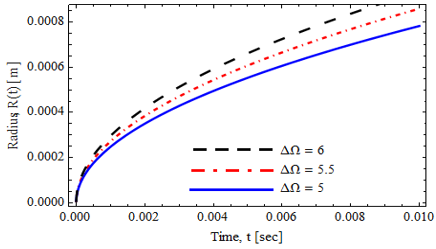Figure 4. Bubble radius growth R(t) with time t for different values of superheating Ω of Al2O3/H2O nanofluids

## 5.png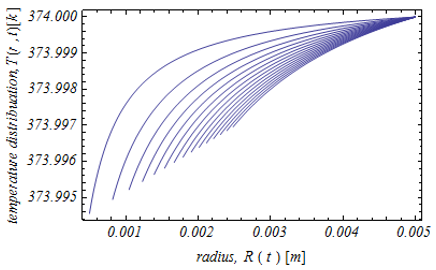(5A)) $\chi=0.1$

## 5b.png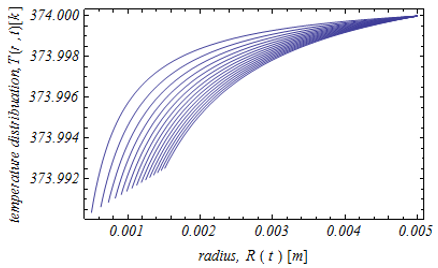(5B)) $\chi=0.5$

Figure 5. Temperature distribution around a growing bubble in Fe3O4/H2O nanofluids for some different values of nanoparticles volume concentration $\chi$

Figure 4 shows the bubble growth for some different values of superheating of nanofluids. We observed that the bubble radius is increasing when the superheating of nanofluids increases.

Figure 5 reveals the influence of nanoparticle volume concentration $\chi$ on the temperature distribution around the growing of bubble in Al2O3/H2O nanofluids where we note that the temperature distribution is proportional with $\chi$. Figure 6 illustrates the temperature distribution around a growing bubble in Fe3O4/H2O, CuO//H2O, Al2O3/H2O nanofluids and water fluid in the turbulent flow. We observed the temperature distribution surrounding the bubbly flow in water is more intensive than in other cases of nanoparticles nanofluids.

## 6a.png(6A) $\mathrm{Al}_{2} \mathrm{O}_{4} / \mathrm{H}_{2} \mathrm{O}$ nanofluids

## 6b.png(6B) CuO//H2O nanofluids

## 6c.png(6C) Fe3O4/H2O nanofluids

## 6d.png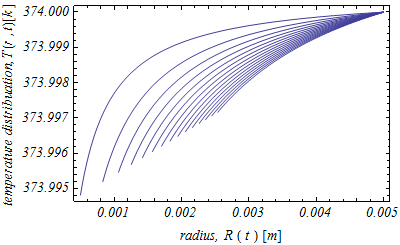(6D) pure water

Figure 6. Temperature distribution around a growing bubble wthin some different nanoparticles/ water nanofluid and pure water

The comparison between the present model in nanofluids and other published models [22, 24] in the cases turbulent flow are shown in Figure 7. Moreover, Figure 8 shows also the comparison between current model and previous studies [11, 20, 21, 31] in laminar flow and pure water. Our results agree with the results of the model  in the case nanoparticle/water nanofluids. In final, we conclude the behavior of bubble growth in nanoparticles/water nanofluids is lower than in the case of pure water in turbulent flow under the influence of heat transfer. Besides, the given results demonstrate that the growth of vapour bubble in laminar flow is bigger than in turbulent flow. The presented results are the agreement with the theoretical study in ref.  in turbulent flow.

## 7.png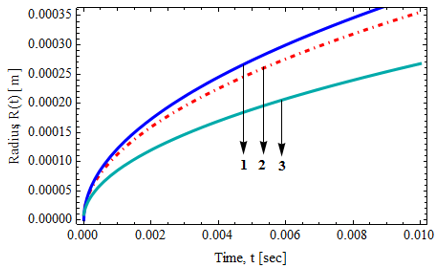Figure 7. Comparison the present model in $\mathrm{Fe}_{3} \mathrm{O}_{4} / \mathrm{H}_{2} \mathrm{O}$ nanofluids with the previous studies in refs. [22, 24] in turbulent flow. Curve 1- results in ref. , curve 2- results in ref.  and curve 3- presented results in equation (28)

## 8.png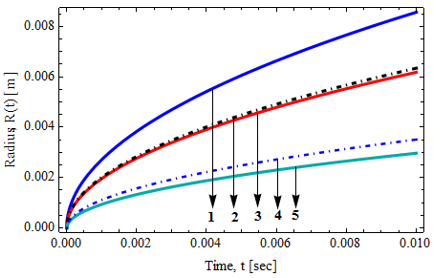Figure 8. Comparison between the current model and some of previous works in the case of laminar flow. Curve 1- results by Scriven model , curve 2- results by Plesset and Zwick model , curve 3- results by Mohammadein and Gouda model , curve 4-results by Abu-Nab et al. , and curve 5- given solution in equation (28); for Ck=1

5. Conclusions

We present the results of the influence of heat transfer on bubble dynamics with some different types of nanofluids like $\mathrm{Fe}_{3} \mathrm{O}_{4} / \mathrm{H}_{2} \mathrm{O}, \mathrm{CuO} / \mathrm{H}_{2} \mathrm{O}, \mathrm{Al}_{2} \mathrm{O}_{3} / \mathrm{H}_{2} \mathrm{O}$ and water fluid between a two-phase turbulent flow. The results show that the effect of nanoparticles volume concentration in nanofluids reduces the growth process under the effect of heat transfer. The radius of vapour bubble in nanoparticles/water nanofluids is less than in pure water. The temperature distribution surrounding the regime of bubble growth in water is more intensive than in other cases of nanoparticles/water $\left(\mathrm{Al}_{2} \mathrm{O}_{3} / \mathrm{H}_{2} \mathrm{O}, \mathrm{Fe}_{3} \mathrm{O}_{4} / \mathrm{H}_{2} \mathrm{O},\right.$ and $\left.\mathrm{CuO} / \mathrm{H}_{2} \mathrm{O}\right)$ nanofluids. Besides, the given results demonstrate that the bubble radius in turbulent flow is less than in turbulent flow. The obtained results of bubble growth in the nanofluids are agreement with the results of previous studies [11, 21, 22, 24, 31] at special cases of turbulent flow and laminar flow. This conclusion must be taken into account in the field of the nuclear power system and other applications of bubbles field.

Nomenclature
 a, b arbitrary constants in Eq. (2) al thermal diffusivity Ck constant defined by Eq. (3) Cp specific heat, $\left(\mathrm{J} \mathrm{kg}^{-1} \mathrm{~K}^{-1}\right)$ c0 constant defined by Eq. (4) L latent heat, $\left(\mathrm{Jkg}^{-1}\right)$ n constant in Eq. (1) V velocity vector in mixture $\left(m s e c^{-1}\right)$ pe Péclet number r radial coordinate R radius of bubble (m) $\dot{R}$ velocity of bubble $\left(\left(m s e c^{-1}\right)\right.$ T temperature (K) s parameter defined by in (7) t time (sec) Greek symbols $k_{l}$ thermal conductivity, $\left.\left(\mathrm{Wm}^{-1} \mathrm{~K}^{-1}\right)\right)$ $\mathcal{A}$ arbitrary constants in Eq. (26) $\sigma$ surface tension $\left(\mathrm{Kg} \mathrm{sec}^{-2}\right)$ $\rho$ density $\left(\operatorname{Kg} \mathrm{m}^{-3}\right)$ $\beta$ parameter defined in (7) $\Delta \Omega$ superheating temperature (K) $J_{a}$ Jacob number $\chi$ nanoparticles volume concentration $\phi_{0}$ void fraction $\epsilon$ ratio of density Subscripts 0 initial value m maximum value Lam laminar flow Tur turbulent flow v vapour nf nanofluid l Liquid f fluid p particle
References

 Choi, S.U.S., Eastman. J.A. (1995). Enhancing thermal conductivity of fluids with nanoparticles. ASME International Mechanical Engineering Congress and Exposition, 66: 99-I05. https://doi.org/W-31109-ENG-38

 Kim, S.J., McKrell, T., Buongiorno, J., Hu, L. (2010). Subcooled flow boiling heat transfer of dilute alumina, zinc oxide, and diamond nanofluids at atmospheric pressure. Nuclear Engineering and Design, 240: 1186-1194. https://doi.org/10.1016/j.nucengdes.2010.01.020

 Rana, K.B., Rajvanshi, A.K., Agrawal, G.D. (2013). A visualization study of flow boiling heat transfer with nanofluids. Journal of Visualization, 16: 133-143. https://doi.org/10.1007/s12650-013-0161-6

 Wen, D., Lin, G., Vafaei, S., Zhang, K. (2009). Review of nanofluids for heat transfer applications. Particuology, 7: 141-150. https://doi.org/10.1016/j.partic.2009.01.007

 Wang, Y., Wu, J. (2016). Single bubble dynamic behavior in AL2O3/H2O nanofluid on downward-facing heating surface. Nuclear Engineering and Technology, 48: 915-924. https://doi.org/10.1016/j.net.2016.02.008

 Saidur, R., Leong, K.Y., Mohammad, H.A. (2011). A review on applications and challenges of nanofluids. Renewable and Sustainable Energy Reviews, 15: 1646-1668. https://doi.org/10.1016/J.RSER.2010.11.035

 Mohammadein, S.A., Abu-Nab, A.K., Shalaby, G.A. (2017). The behavior of vapour bubbles inside a vertical cylindrical tube under the effect of peristaltic motion with two-phase density flow and heat transfer. Journal of Nanofluids, 6(5): 940-945. https://doi.org/10.1166/jon.2017.1385

 Khanafer, K., Vafai, K. (2012). A critical synthesis of thermo physical characteristics of nanofluids. International Journal of Heat and Mass Transfer, 54: 4410-442. https://doi.org/10.1016/j.ijheatmasstransfer.2011.04.048

 Sajadifar, S.A., Karimipour, A., Toghraie, D. (2017). Fluid flow and heat transfer of non Newtonian nanofluid in a micro tube considering slip velocity and temperature jump boundary conditions. European Journal Mechanics B Fluids, 61: 25-32. https://doi.org/10.1016/j.euromechflu.2016.09.014

 Parsaiemehr, M., Pourfattah, F., Akbar, O.A., Toghraie, D., Sheikhzadeh, G. (2018). Turbulent flow and heat transfer of Water/Al2O3 nanofluid inside a rectangular ribbed channel. Physica E: Low-dimensional Systems and Nanostructures, 96: 73-84. https://doi.org/10.1016/j.physe.2017.10.012

 Scriven, L.E. (1959). On the dynamics of phase growth. Chemical Engineering Science, 10(1-2): 1-13. https://doi.org/10.1016/0009-2509(59)80019-1

 Park, H., Lee, S.J., Jung S.Y. (2019). Effect of nanofluid formation methods on behaviors of boiling bubbles. International Journal of Heat and Mass Transfer, 135: 1312-1318. https://doi.org/10.1016/j.ijheatmasstransfer.2019.02.091

 Bhatt, D., Kangude, P., Srivastava, A. (2019). Simultaneous mapping of single bubble dynamics and heat transfer rates for SiO2/water nanofluids under nucleate pool boiling regime. Physics of Fluids, 31: 017102. https://doi.org/10.1063/1.5050980

 Goel, P., Nayaka, A.K., Kulkarni, P.P., Joshi, J.B. (2017). Experimental study on bubble departure characteristics in subcooled nucleate pool boiling. International Journal of Multiphase Flow, 89: 163-176. https://doi.org/10.1080/08916152.2017.1397821

 Fan, L., Hemminger, O., Yu, Z., Wang F. (2007). Bubbles in nanofluids. Industrial and Engineering Chemistry Research, 46(12): 4341-4346. https://doi.org/10.1021/ie061532c

 Tanasawa, I., Yang, W.J. (1970). Dynamic behavior of a gas bubble in visco-elastic liquids. Journal of Applied Physics, 41(11): 4526-4531. https://doi.org/10.1063/1.1658491

 Ting, R.Y. (1975). Visco-elastic effect of polymers on single bubble dynamics. AIChE Journal, 21(4): 810-813. https://doi.org/10.1002/aic.690210427

 Mohammadein, S.A., Shalaby, G.A., Abu-Bakr, A.F., Abu-Nab, A.K. (2017). Analytical solution of gas bubble dynamics between two-phase flow. Results in Physics, 7: 2396-2403. https://doi.org/10.1016/j.rinp.2017.07.007

 Abu-Bakr, A.F., Abu-Nab, A.K. (2021). Vapour bubble growth within a viscous mixture non-Newtonian fluid between two-phase turbulent flow. International Journal of Ambient Energy. https://doi.org/10.1080/01430750.2021.1875046

 Abu-Nab, A.K., Selima E.S., Morad A.M. (2021). Theoretical investigation of a single vapor bubble during Al2O3/H2O nanofluids in power-law fluid affected by a variable surface tension. Physica Scripta, 96: 035222. https://doi.org/10.1088/1402-4896/abdb5a

 Plesset, M., Zwick, S. (1954). The growth of vapour bubbles in superheated liquids. Journal of Applied Physics, 25: 493-500. https://doi.org/10.1063/1.1721668

 Mohammadein, S.A., Abu-Bakr, A.F. (2010). The growth of vapour bubble in a superheated liquid between two-phase turbulent flow. Canadian Journal of Physics, 88(5): 317-324. https://doi.org/10.1139/P10-022

 Batchelor, G.K. (1959). The Theory of Homogeneous Turbulence. Cambridge University Press. London. https://doi.org/10.1146/annurev.fluid.34.081701.13482

 Abu-Bakr, A.F. (2019). Theory of bubble dynamics: temperature distribution around the growing bubbles of turbulent flow. Journal of Nanofluids, 8(2): 371-378. https://doi.org/10.1166/jon.2019.1592

 Borishanskii, V.M., Zebloiskaya, V. (1967). Turbulent heat transfer of a liquid with high thermal conductivity. Atomnaya Energiya, 22(2): 147-149. https://doi.org/10.1007/BF01246281

 Wang, Y., Wu, J.M. (2015). Numerical simulation on single bubble behavior during Al2O3/H2O nanofluids flow boiling using moving particle simi-implicit method. Progress in Nuclear Energy, 85: 130-139. https://doi.org/10.1016/J.PNUCENE.2015.06.017

 Koo, J., Kleinstreuer, C. (2004). A new thermal conductivity model for nanofuids. Journal of Nanoparticle Research, 6(6): 577-588. https://doi.org/10.1007/s11051-004-3170-5

 Sayed, A.Y., Abdel-wahed, M.S. (2020). Entropy analysis for an MHD nanofluid with a microrotation boundary layer over a moving permeable plate. Eurpean Physics Journal Plus, 135: 106. https://doi.org/10.1140/epjp/s13360-020-00181-6

 Abdel-wahed, M.S. (2019). Magnetohydrodynamic Ferro-Nano fluid flow in a semi-porous curved tube under the effect of hall current and nonlinear thermal radiative. Journal of Magnetism and Magnetic Materials, 474: 347-354. https://doi.org/10.1016/j.jmmm.2018.11.050

 Haar, L., Callagher, J.S., Kell, G.S. (1984). NBS/NRV, Steam tables. https://doi.org/10.1002/cite.330570931

 Mohammadein, S.A., Gouda, S.A. (2006). Temperature distribution in a mixture surrounding a growing vapour bubble. Heat Mass Transfer, 42(5): 359-363. https://doi.org/10.1007/s00231-004-0585-6